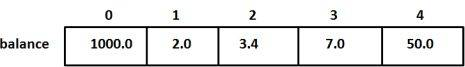# C++ 数组

## C++ 数组

C++ 支持数组数据结构，它可以存储一个固定大小的相同类型元素的顺序集合。数组是用来存储一系列数据，但它往往被认为是一系列相同类型的变量。

## 声明数组

```type arrayName [ arraySize ];
```

```double balance;
```

## 初始化数组

```double balance = {1000.0, 2.0, 3.4, 17.0, 50.0};
```

```double balance[] = {1000.0, 2.0, 3.4, 17.0, 50.0};
```

```balance = 50.0;
```## 访问数组元素

```double salary = balance;
```

``````#include <iostream>
using namespace std;

#include <iomanip>
using std::setw;

int main ()
{
int n[ 10 ]; // n 是一个包含 10 个整数的数组

// 初始化数组元素
for ( int i = 0; i < 10; i++ )
{
n[ i ] = i + 100; // 设置元素 i 为 i + 100
}
cout << "Element" << setw( 13 ) << "Value" << endl;

// 输出数组中每个元素的值
for ( int j = 0; j < 10; j++ )
{
cout << setw( 7 )<< j << setw( 13 ) << n[ j ] << endl;
}

return 0;
}``````

```Element        Value
0          100
1          101
2          102
3          103
4          104
5          105
6          106
7          107
8          108
9          109
```

## 您可能还喜欢：

App下载